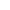Top | Introduction | Members | Activities | Call for Paper | Link |Japanese## "Reserch for Countermeasure of Side Channel Analysis on Elliptic Curve Cryptography"

 The public key cryptosystem is different encryption key whish is public from decryption key which is secret. It is used for not only encryption but also the e-signature. By the improvement of decipher, RSA cryptosystem and Elgamal cryptosystem that is a past public key cryptosystem. Safety is threatened. An elliptic curve cryptosystem (ECC) and a hyper-elliptic curve cryptosystem (HECC) are actively researched for that as next generation's cryptosystems. The elliptic curve cryptosystem is based on the points on an elliptic curve becoming a group, and elliptic discrete logarithm problem. It was proposed by Koblitz and Miller. Encryption and decryption Key size of ECC is short and compactly compared with RSA etc. Therefore, it is used from this feature with the smart card with a small memory.The operation that becomes the center of the elliptic curve cryptosystem is an operation of scalar multiplication $dP$ to point $P$ on an elliptic curve and secret key $d$ regardless of decoding or the signature generation of the ciphertext. It is a side channel attack that becomes a threat because of implementation the smart card of ECC. The side channel attack is an attack to obtain the secret key by using side channel information on power consumption and the execution time etc. Generally, when conputing the scalar multiplication $dP$, the binary method is executed. In the operation, because $x$ is expressed by the bit string, The power consumption is differnt between bit is 1 and 0. Thus the secret key leaks by the power consumption. Therefore, countermeasures of the side channel attack have been researched. The side channel attacks are classified simple power analysis attack (SPA) and different power analysis analysis (DPA). SPA is an attack of the pattern of waves of the power consumption under the operation to obtain the secret key by one observation. It is necessary to lose the dependence of the confidential information and power consumption to prevent SPA. Existing countermeasures method inserts the dummy operation while operating it, and includes the method of making the pattern of waves constant. It is DPA that the attacker simulate the test key and taking the difference of power consumption with a true secret key. In DPA, it is classified into Data-bit DPA(DDPA) and Address-bit DPA(ADPA). There are DDPA countermeasures that includes the method of using the randomization scalar and a random point, and ADPA measures have the method of making the randomization scalar and concealing the relation between the scalar and the address. However, both computational complexities and the memories tend to increase by existing measures method to SPA, DDPA, and ADPA. Moreover, there is a problem that each measures method is safe only in a each attack method. In this research, I proposed effective algorithms of scalar multiplicationthis which are countermeasure on SPA, DDPA, ADPA. The window method is used as a scalar multiplication algorithm in this research. The window method prepare preliminary operation table. Using the table, it is used can high-speed computation. In the proposal method, it proposes DDPA countermeasures and ADPA countermeasures in the window method. In DDPA countermeasures, the method of the randomization scalar is used. For window method when applied SPA countemeasure , Table size increase becaouse using fix window. And by DDPA countermeasure, computational complexity by table making increases by using random point and data. In proposed scheme, I apply Side channel atomicity to an SPA countermeasure and, by DDPA countermeasure, use a method to do randomisation of a scalar. the randomizing of the scalar is done by deciding whether to execute the conversion of the expression of each operated window at random by using becoming $(100\bar{8}) _ 2=0(\bar{8}=-8)$. By this proposec scheme, randomisation of scalar expression is possible. Furthermore, I give presence of conversion with a random number in the case of 0bit so that increase the number of the randomisation. Usually, Last bit of bit string in window execute only doubling in the case of 0 bit by window method, but execute presence of the insertion of the addition that does not influence a scalar value in 0bit either by a random number by proposed method. By choice of processing by 0bit time, the number of the randomisation increases more. I really sacrificed computational complexity, but the number of the randomisation increased than randomisation technique of existing scalar expression. ADPA measures use the method of concealing the relation between the scalar and the address. Because the relation between data and the address of the table is rotated at random, the relation is concealed. Both countereasures are given without the computational complexity increasing and addition one memory. And I proposed the window method algorithm that considered SPA measures in addition to these measures.  [ back ]